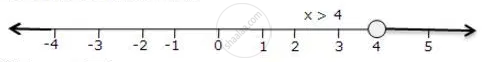Share

Books Shortlist

# Represent the Solution of the Given Inequalities on the Real Number Line 4x - 1 > X + 11 - ICSE Class 10 - Mathematics

ConceptLinear Inequations in One Unknown

#### Question

Represent the solution of the given inequalities on the real number line

4x - 1 > x + 11

#### Solution

4x - 1 > x + 11

3x > 12

x > 4

The solution on number line isIs there an error in this question or solution?

#### APPEARS IN

Solution Represent the Solution of the Given Inequalities on the Real Number Line 4x - 1 > X + 11 Concept: Linear Inequations in One Unknown.
S